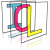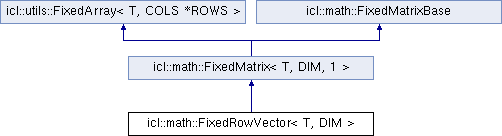Image Component Library (ICL)
icl::math::FixedRowVector< T, DIM > Struct Template Reference

`#include <FixedVector.h>`

Inheritance diagram for icl::math::FixedRowVector< T, DIM >:## Public Types

typedef FixedMatrix< T, DIM, 1 > superPublic Types inherited from icl::math::FixedMatrix< T, DIM, 1 >
typedef T * iterator
iterator type More...

typedef const T * const_iterator
const iterator type More...

typedef T * row_iterator
row_iterator More...

typedef const T * const_row_iterator
const row_iterator More...

typedef const col_iterator const_col_iterator

## Public Member Functions

FixedRowVector ()

FixedRowVector (const T &init)

FixedRowVector (const T *srcData)

FixedRowVector (const FixedMatrix< T, DIM, 1 > &other)

FixedRowVector (const T &v0, const T &v1, const T &v2=0, const T &v3=0, const T &v4=0, const T &v5=0, const T &v6=0, const T &v7=0, const T &v8=0, const T &v9=0, const T &v10=0, const T &v11=0)

template<class Iterator >
FixedRowVector (const FixedMatrixPart< T, DIM, Iterator > &r)

template<class otherT , class Iterator >
FixedRowVector (const FixedMatrixPart< otherT, DIM, Iterator > &r)Public Member Functions inherited from icl::math::FixedMatrix< T, DIM, 1 >
DynMatrix< T > dyn ()
creates a shallow copied DynMatrix instance wrapping this' data More...

const DynMatrix< T > dyn () const
creates a shallow copied DynMatrix instance wrapping this' data (const) More...

FixedMatrix ()
Default constructor. More...

FixedMatrix (const T &initValue)
Create Matrix and initialize elements with given value. More...

FixedMatrix (const T *srcdata)
Create matrix with given data pointer (const version) More...

FixedMatrix (const T &v0, const T &v1, const T &v2=0, const T &v3=0, const T &v4=0, const T &v5=0, const T &v6=0, const T &v7=0, const T &v8=0, const T &v9=0, const T &v10=0, const T &v11=0, const T &v12=0, const T &v13=0, const T &v14=0, const T &v15=0)
Create matrix with given initializer elements (16 values max) More...

FixedMatrix (OtherIterator begin, OtherIterator end)
Range based constructor for STL compatiblitiy. More...

FixedMatrix (const FixedMatrix &other)

FixedMatrix (const FixedMatrix< otherT, COLS, ROWS > &other)

FixedMatrix (const FixedMatrixPart< T, DIM, Iterator > &r)
Create matrix of a sub-part of another matrix (identical types) More...

FixedMatrix (const FixedMatrixPart< otherT, DIM, Iterator > &r)
Create matrix of a sub-part of another matrix (compatible types) More...

FixedMatrixoperator= (const FixedMatrix &other)
Assignment operator (with compatible data type) (deep copy) More...

FixedMatrixoperator= (const FixedMatrix< otherT, COLS, ROWS > &other)
Assignment operator (with compatible data type) (deep copy) More...

FixedMatrixoperator= (const T &t)
Assign all elements with given value. More...

FixedMatrixoperator= (const FixedMatrixPart< T, DIM, Iterator > &r)
Assign matrix elements with sup-part of another matrix (identical types) More...

FixedMatrixoperator= (const FixedMatrixPart< otherT, DIM, Iterator > &r)
Assign matrix elements with sup-part of another matrix (compatible types) More...

FixedMatrix operator/ (const FixedMatrix &m) const
Matrix devision. More...

FixedMatrix operator/ (T f) const
Divide all elements by a scalar. More...

FixedMatrixoperator/= (const FixedMatrix &m)
Matrix devision (inplace) More...

FixedMatrixoperator/= (T f)
Divide all elements by a scalar. More...

FixedMatrix operator * (T f) const
Multiply all elements by a scalar. More...

FixedMatrix< T, MCOLS, ROWS > operator * (const FixedMatrix< T, MCOLS, COLS > &m) const
Matrix multiplication (essential) More...

FixedMatrixoperator *= (T f)
moved outside the class Multiply all elements by a scalar (inplace) More...

FixedMatrix operator+ (const T &t) const
Add a scalar to each element. More...

FixedMatrix operator+ (const FixedMatrix &m) const

FixedMatrixoperator+= (const T &t)
Add a scalar to each element (inplace) More...

FixedMatrixoperator+= (const FixedMatrix &m)

FixedMatrix operator- (const T &t) const
Substract a scalar from each element. More...

FixedMatrix operator- (const FixedMatrix &m) const
Element-wise matrix subtraction. More...

FixedMatrix operator- () const
Prefix - operator. More...

FixedMatrixoperator-= (const T &t)
Substract a scalar from each element (inplace) More...

FixedMatrixoperator-= (const FixedMatrix &m)
Element-wise matrix subtraction (inplace) More...

T & operator() (unsigned int col, unsigned int row)
Element access operator. More...

const T & operator() (unsigned int col, unsigned int row) const
Element access operator (const) More...

T & at (unsigned int col, unsigned int row)
Element access index save (with exception if index is invalid) More...

const T & at (unsigned int col, unsigned int row) const
Element access index save (with exception if index is invalid) (const) More...

T & operator[] (unsigned int idx)
linear data view element access More...

const T & operator[] (unsigned int idx) const
linear data view element access (const) More...

T * data ()
return internal data pointer More...

const T * data () const
return internal data pointer (const) More...

iterator begin ()
returns an iterator to first element iterating over each element (row-major order) More...

const_iterator begin () const
returns an iterator to first element iterating over each element (row-major order) (const) More...

iterator end ()
returns an iterator after the last element More...

const_iterator end () const
returns an iterator after the last element (const) More...

col_iterator col_begin (unsigned int col)
returns an iterator iterating over a certain column More...

const_col_iterator col_begin (unsigned int col) const
returns an iterator iterating over a certain column (const) More...

col_iterator col_end (unsigned int col)
row end iterator More...

const_col_iterator col_end (unsigned int col) const
row end iterator const More...

row_iterator row_begin (unsigned int row)
returns an iterator iterating over a certain row More...

const_row_iterator row_begin (unsigned int row) const
returns an iterator iterating over a certain row (const) More...

row_iterator row_end (unsigned int row)
row end iterator More...

const_row_iterator row_end (unsigned int row) const
row end iterator (const) More...

void mult (const FixedMatrix< T, MCOLS, COLS > &m, FixedMatrix< T, MCOLS, ROWS > &dst) const
inplace matrix multiplication (dst = (*this)*m) More...

FixedMatrix inv () const
invert the matrix (only implemented with IPP_OPTIMIZATION and only for icl32f and icl64f) More...

det () const
calculate matrix determinant (only implemented with IPP_OPTIMIZATION and only for icl32f and icl64f) More...

FixedMatrix< T, ROWS, COLS > transp () const
returns matrix's transposed More...

element_wise_inner_product (const FixedMatrix< T, OTHER_COLS, DIM/OTHER_COLS > &other) const
inner product of data pointers (not matrix-mulitiplication) More...

FixedMatrix< T, OTHER_COLS, COLS > dot (const FixedMatrix< T, OTHER_COLS, ROWS > &M) const
returns the inner product of two matrices (i.e. dot-product) More...

double cond (const double p=2) const
computes the condition of a fixed matrix More...

trace () const
computes the sum of all diagonal elements More...

FixedMatrixPart< T, COLS, row_iteratorrow (unsigned int idx)
returns a matrix row-reference iterator pair More...

FixedMatrixPart< T, COLS, const_row_iteratorrow (unsigned int idx) const
returns a matrix row-reference iterator pair (const) More...

FixedMatrixPart< T, ROWS, col_iterator > col (unsigned int idx)
returns a matrix col-reference iterator pair More...

FixedMatrixPart< T, ROWS, const_col_iteratorcol (unsigned int idx) const
returns a matrix col-reference iterator pair (const) More...

FixedMatrixPart< T, WIDTH *HEIGHT, MatrixSubRectIterator< T > > part ()
extracts a rectangular matrix sub region More...

const FixedMatrixPart< T, WIDTH *HEIGHT, MatrixSubRectIterator< T > > part () const
extracts a rectangular matrix sub region (const) More...

FixedMatrix< T, NEW_WIDTH, NEW_HEIGHT > resize (const T &init=T(0)) const
extends/shrinks matrix dimensions while preserving content on remaining elements (without scaling) More...

double length (T norm=2) const
Calculates the length of the matrix data vector. More...

void normalize (T norm=2)
inplace normalization More...

FixedMatrix< T, COLS, ROWS > normalized (T norm=2) const
create a normalized version of this matrix More...

bool operator== (const FixedMatrix< otherT, COLS, ROWS > &m) const
Element-wise comparison with other matrix. More...

bool operator!= (const FixedMatrix< otherT, COLS, ROWS > &m) const
Element-wise comparison with other matrix. More...

FixedMatrix< T, 1, ROWS > diag () const
returns a vector of the diagonal elements (only for squared matrices) More...

void decompose_QR (FixedMatrix< T, COLS, ROWS > &Q, FixedMatrix< T, COLS, COLS > &R) const
computes the QR decomposition of a matrix More...

void decompose_RQ (FixedMatrix< T, ROWS, ROWS > &R, FixedMatrix< T, ROWS, ROWS > &Q) const
computes the RQ decomposition of a matrix More...

void svd (FixedMatrix< T, COLS, ROWS > &U, FixedMatrix< T, 1, COLS > &s, FixedMatrix< T, COLS, COLS > &V) const
computes Singular Value Decomposition of this Matrix A = U diag(s) V' More...

FixedMatrix< T, ROWS, COLS > pinv (bool useSVD=0, float zeroThreshold=0.00000000000000001) const
Computes the Matrix's pseudo-inverse. More...

void eigen (FixedMatrix &eigenvectors, FixedMatrix< T, 1, COLS > &eigenvalues) const
Extracts the matrix's eigenvalues and eigenvectors. More...Public Member Functions inherited from icl::utils::FixedArray< T, COLS *ROWS >
T & operator[] (unsigned int idx)
index access operator More...

const T & operator[] (unsigned int idx) const
index access operator (const) More...Static Public Member Functions inherited from icl::math::FixedMatrix< T, DIM, 1 >
static const FixedMatrixnull ()
returning a reference to a null matrix More...

static unsigned int rows ()
compatibility-function returns template parameter ROWS More...

static unsigned int cols ()
compatibility-function returns template parameter COLS More...

static unsigned int dim ()
return static member variable DIM (COLS*ROWS) More...

static FixedMatrix< T, ROWS, COLS > id ()
create identity matrix More...Static Public Member Functions inherited from icl::math::FixedMatrixBase
template<class SrcIterator , class DstIterator , unsigned int N>
static void optimized_copy (SrcIterator srcBegin, SrcIterator srcEnd, DstIterator dstBegin)
Optimized copy function template (for N>30 using std::copy, otherwise a simple loop is used) More...Public Attributes inherited from icl::utils::FixedArray< T, COLS *ROWS >
m_data [DIM]Static Public Attributes inherited from icl::math::FixedMatrix< T, DIM, 1 >
static const unsigned int DIM
count of matrix elements (COLS x ROWS) More...

## ◆ super

template<class T , int DIM>
 typedef FixedMatrix icl::math::FixedRowVector< T, DIM >::super

## ◆ FixedRowVector() [1/7]

template<class T , int DIM>
 icl::math::FixedRowVector< T, DIM >::FixedRowVector ( )
inline

## ◆ FixedRowVector() [2/7]

template<class T , int DIM>
 icl::math::FixedRowVector< T, DIM >::FixedRowVector ( const T & init )
inlineexplicit

## ◆ FixedRowVector() [3/7]

template<class T , int DIM>
 icl::math::FixedRowVector< T, DIM >::FixedRowVector ( const T * srcData )
inlineexplicit

## ◆ FixedRowVector() [4/7]

template<class T , int DIM>
 icl::math::FixedRowVector< T, DIM >::FixedRowVector ( const FixedMatrix< T, DIM, 1 > & other )
inline

## ◆ FixedRowVector() [5/7]

template<class T , int DIM>
 icl::math::FixedRowVector< T, DIM >::FixedRowVector ( const T & v0, const T & v1, const T & v2 = `0`, const T & v3 = `0`, const T & v4 = `0`, const T & v5 = `0`, const T & v6 = `0`, const T & v7 = `0`, const T & v8 = `0`, const T & v9 = `0`, const T & v10 = `0`, const T & v11 = `0` )
inline

## ◆ FixedRowVector() [6/7]

template<class T , int DIM>
template<class Iterator >
 icl::math::FixedRowVector< T, DIM >::FixedRowVector ( const FixedMatrixPart< T, DIM, Iterator > & r )
inline

## ◆ FixedRowVector() [7/7]

template<class T , int DIM>
template<class otherT , class Iterator >
 icl::math::FixedRowVector< T, DIM >::FixedRowVector ( const FixedMatrixPart< otherT, DIM, Iterator > & r )
inline

The documentation for this struct was generated from the following file: